# My journey on the Giscup 2015

Organizers of the 23rd ACM SIGSPATIAL International Conference on Advances in Geographic Information Systems (ACM SIGSPATIAL 2015) have challenged the GIS community to participate in a coding competition about finding the shortest path (space and time based) under polygonal obstacle constraints.

Shortest path algorithms are widely used today, and they are vital for routing services such as Google Maps, Microsoft Bing or Here.
This competition was focusing on single source single destination shortest path algorithms where the shortest path between two nodes of the graph is the target for the search. The contest also adds difficulties by introducing the polygonal obstacles which mean they generated polygons what should be avoided during the shortest path generation.

The contest masters defined the competition’s target as the calculated shortest path quality and the computation time of calculation (excluding all the I/O operations). Unfortunately, there was no exact definition of the evaluation method they used, but this guideline was enough for the contesters to optimize their approach.

One of the best-known algorithms for this problem (SSSP) is the A* algorithm. I selected this algorithm because the task defined only one shortest path search per run and this algorithm requires no preprocessing as it can be run on the data after it is loaded into the memory. To get the fastest result, I have selected C++ for the implementation language and I tried to avoid overhead on object oriented data structures, so I picked up the adjacency list data structure implemented as simple arrays. The algorithm maintains an inner data structure to pick up a node in every iteration. This data structure must support quick extractMin operation as well as quick insertion method. One of the best available options for this specification is the heap data structure. Studies are suggested to use different heap approaches for the A* algorithm what I wanted to investigate therefore I have included two versions of the heap data structure: binary heap and a 4-ary heap.

The A* algorithm allows us to choose between search directions (forward, backward, bi-directional). To investigate which direction can be the best for the competition, I have implemented all three of them and did a quick in-house evaluation to find out which is the winner approach.

Before I introduce the result, let’s have a look at the example data given by the competition masters. I implemented a quick application to convert the example file into a well-known text format file which then can be loaded into QGIS.

The example file contains a road network with 42408 nodes and 96850 directed links between nodes. The link/node ratio for this graph is something that someone would expect from a graph represents a road network.
There is only one thing what I want to bring into the attention. The practice amongst GIS map digitization people is to build up a curved street with many nodes and links. You can see an example in the following image.

This discovery can give a chance for optimization. When the shortest path algorithm considers its iterations, it is always doing node by nodes. If you consider a long highway which has a long curvature, then when the algorithm considers that highway segment, it has to do many iterations. However, if this segment would be represented by only one link, then the algorithm could evaluate that segment much quicker.
The following example shows what the proposed simplification should achieve.

Applying this simplification gives the following output for the example data file.

After running the simplification algorithm, the road network simplified to 86194 links (-11%). There is, however, an unexpected behavior for this simplification. As you can see in the following image, some of the nodes have been lost their links.

The explanation for this behavior is described by the following image.Details of the previous case. The last piece of the two-way road segment will be removed because it is recognized as a 2-long segment chain

Now everything was ready up for the experimentation. In the first step, I was curious about how the simplification algorithm could help to speed up the search. I have run the complete application which has four different parts (reading files, preprocessing data, shortest path calculation, writing out the results) exactly 10 times to avoid runtime fluctuation caused by the operating system’s task scheduling policy. I have picked up an origin and destination node for the application which seemed to be one of the longest. The simplification is inserted into the phase when the application creates the necessary arrays to run the shortest path algorithm. The simplification itself adds time to the execution time but I expected to have benefit when the search uses the simplified road network.Runtime comparison. The simplification requires approximately 5000ms more time and it only decreases the time of the shortest path calculation by 400ms.

Unfortunately, my quick experiment showed that using the simplification algorithm actually slows down the overall shortest path calculation, because the algorithm couldn’t get more speed up then the extra time caused by the road network simplification. However, if the challenge would be a batch of shortest path calculations (not only one) or the road network would contain more highways then the simplification could have a serious impact on the runtime.
For the second run, I wanted to know what version of the A* algorithm is the quickest to solve the problem. I have run the forward, backward and bidirectional version of the A* with the available two heap implementations (binary heap, 4-ary heap).

According to the literature, the bidirectional A* algorithm should find the shortest path faster than the forward or the backward version. My measurement resulted in the opposite result. The forward implementation required 1362 (length-based) and 541 (time-based) iterations, the backward needed 3453 (length-based) and 977 (time-based), the bidirectional took 1662 (length-based) and 5125 (time-based) iterations. The runtime analysis showed even more difference between the bidirectional and the other versions. The large runtime difference was caused because the bidirectional version requires two heaps (one forward and one backward heap) during the calculation and the data cannot be cached properly however when the algorithm has only one heap, the data can be cached and accessed very quickly. I have run a quick memory usage statistic analyses to find out this is true or not. Valgrind confirmed this behavior because the shortestPathCalculation function caused 5792 (forward), 19464 (backward), 70983 (bidirectional) cache misses (D1mr).

Based on the measurements, I have submitted my application contains the forward A* algorithm using the 4-ary heap plus the introduced road network simplification method. This solution was ranked as 5th valid submission. Unfortunately, the contest masters didn’t give more explanation about their final judging method after publishing the result. For me, it was a great exercise and I have learned a lot. Implementing the most simple GIS method can be very painful using iterative programming languages and algorithms theoretically faster than others behaves differently in practice. Let’s hope that the next years Giscup will be focusing again an interesting problem and I will be able to contribute to that competition as well.

The source code is available on GitHub at the following link.

# Experimenting with Dijkstra’s algorithm

This little project aims to measure the performance of different implementation of one of the most known single source shortest path algorithm, Dijkstra’s shortest path algorithm. I am considering the naive, priority queue with binary heap and priority queue with Fibonacci heap type implementations where I am using existing open-source implementation of the Fibonacci heap. My goal with this experiment is to show different implementation’s strength and also to investigate the performance of existing open-source Fibonacci heap implementations. All the theoretical knowledge can be found in CLRS 24.3 and CLRS 19. To get deeper understanding on the measurement, I have decided to split the text into 4 parts:

• Implementation details
• Data generation and measurement scenarios
• Results

### Dijkstra’s algorithm

Dijkstra’s shortest path algorithm is a single source shortest path algorithm. It is calculating all shortest paths from single source point/vertex/node. The algorithm itself is creating a shortest path spanning tree which is always stored in a vector, called the previous vector. This vector helps us to create the actual shortest path from the origin to the target by stepping back on this vector. For more details, see CLRS 24.3.

All the different versions of Dijkstra’s algorithm differ in the way they are creating the previous vector. The first naive implementation’s pseudo code is the following: (I have used here the pseudocode from Wikipedia, because it is easy to understand)

``````function Dijkstra(Graph, source):
dist  := 0                  // Distance from source to source
prev  := undefined          // Previous node in optimal path initialization

for each vertex v in Graph:         // Initializations
if v ≠ source                     // Where v has not yet been removed from Q (unvisited nodes)
dist[v]  := infinity            // Unknown distance function from source to v
prev[v]  := undefined           // Previous node in optimal path from source
end if
add v to Q                        // All nodes initially in Q (unvisited nodes)
end for

while Q is not empty:               // The main loop
u := vertex in Q with min dist[u] // Source node in first case
remove u from Q

for each neighbor v of u:         // where v has not yet been removed from Q.
alt := dist[u] + length(u, v)
if alt < dist[v]:            // A shorter path to v has been found
dist[v]  := alt
prev[v]  := u
end if
end for
end while

return dist[], prev[]

end function```
```

This is going to be my first, naive implementation of the Dijkstra’s algorithm where I am using a simple full scan search for finding the closest vertex in the distance vector. This comes with O(n) time complexity so the overall time complexity is O(|E| + |V|2). It is clear that finding the closest vertex/node can be achieved by maintaining an advanced data structure to reduce the search time. Priority Queues can help in this, where the queue can be implemented as a binary heap reducing time complexity to O((|E|+|V|)log|V|) or a more advanced data structure developed specially for this algorithm, the Fibonacci heap where the time complexity can be reduced to O(|E| + |V|log|V|). The code for calculating the previous vector in general is the following:

``````function Dijkstra(Graph, source):
dist := 0                 // Initializations
for each vertex v in Graph:
if v ≠ source
dist[v] := infinity           // Unknown distance from source to v
previous[v] := undefined      // Predecessor of v
end if
end for

while Q is not empty:             // The main loop
u := Q.extract_min()            // Remove and return best vertex
mark u as scanned

for each neighbor v of u:
if v is not yet scanned:
alt = dist[u] + length(u, v)
if alt < dist[v]
dist[v] := alt
previous[v] := u
Q.decrease_priority(v,alt)
end if
end if
end for
end while

return previous[]
end function```
```

Using Fibonacci heap for the Priority Queue can be decrease the running time, because it has θ(1) amortized cost on Decrease key and insert operations compared to the θ(lgn) Decrease key operation of binary heap. The following table is showing all the necessary operations for the Priority Queue implementation of both heap (CLRS 19.1):

Procedure Binary heap Fibonacci heap
MAKE-HEAP θ(1) θ(1)
INSERT θ(lgn) θ(1)
MINIMUM θ(1) θ(1)
EXTRACT-MIN θ(lgn) O(lgn)
DECREASE-KEY θ(lgn) θ(1)
DELETE θ(lgn) O(lgn)

The Fibonacci heap itself was developed based on the observation that usually there are multiple Decrease-Key operations related to one Extract-Min operation.

### Implementation details

In this framework, there are a couple of different implementations of the algorithm, but they can be classified into three different groups:

• Naive implementation
• Binary Tree Priority Queue implementation
• Fibonacci Heap Priority Queue implementation

Before I introduce the implementation details of the algorithms, I will introduce how the framework is implementing the graph. I coded the neighbourhood list approach where two nested arrays represent the actual graph: there is a int[][] which is storing the neighbour nodes for each node and a double[][] which is storing the corresponding arc/edge weights.

##### Naive implementation

It is obviously the slowest version (algorithmically), however the results of this version can be useful for validating the other implementation correctness. Also it is interesting to see how this simple version is performing against other advanced implementation. The simplicity of this implementation is giving the chance to the Java VM to optimize the code and also it is giving the opportunity to the hardware architecture for low-level optimization (for example branch prediction).

The actual implementation can be found here: BaseDijsktra

##### Binary Tree Priority Queue implementation

As mentioned before, it is possible to use Priority Queues to speed up the algorithm. For this and the other Priority Queue style algorithms, I created a general code to test them. This version of the Dijkstra can be found here: PriorityQueueDijkstra For every single PriorityQueue algorithm, there is a wrapper class which is using the heap algorithm inside to represent the Priority Queue. The interface for it can be found here: PriorityQueue. There is a built-in binary search tree (TreeSet, which is based on TreeMap which is a red-back binary search tree) in Java and the framework uses this search tree for the Priority Queue.

Implementation of the TreeSet Priority Queue can be found here: TreeSetPriorityQueue

##### Fibonacci Heap Priority Queue implementation

Instead of trying to implement myself the Fibonacci heap, I tried to find existing open-source implementations. After reading through many pages of the Google search “Fibonacci heap Java” I could find the following ones:

As mentioned before, I created individual wrapper classes for the different implementation of Priority Queues:

### Data generation and measurement scenarios

For the data generation, I have created a random graph generator. This generator created random directed graphs according the two parameters:

• size of the graph in the terms of vertices/nodes in the graph (parameter n)
• connectivity of the graph: how sparse is the graph (parameter p)
• the connectivity is expressed by a 0.0 <= p <= 1.0 floating number where 0.0 means there is no edge/arc in the graph and 1.0 means it is a complete graph
• Here is just a simple example of the graph generation method with different connectivity parameter: (online version of the Graphviz) was used to generate these graph using the output of this simple program: GraphGeneratorDotOutputMain, Output text file)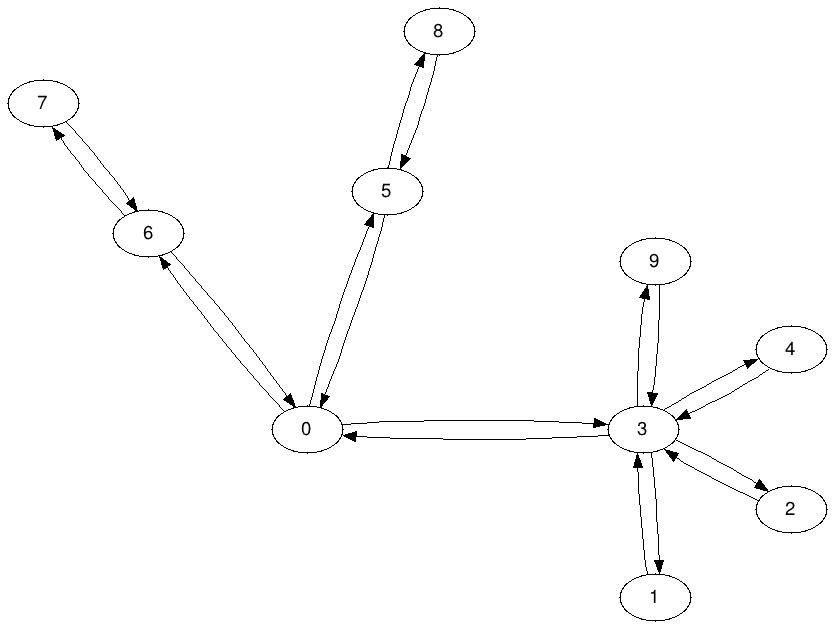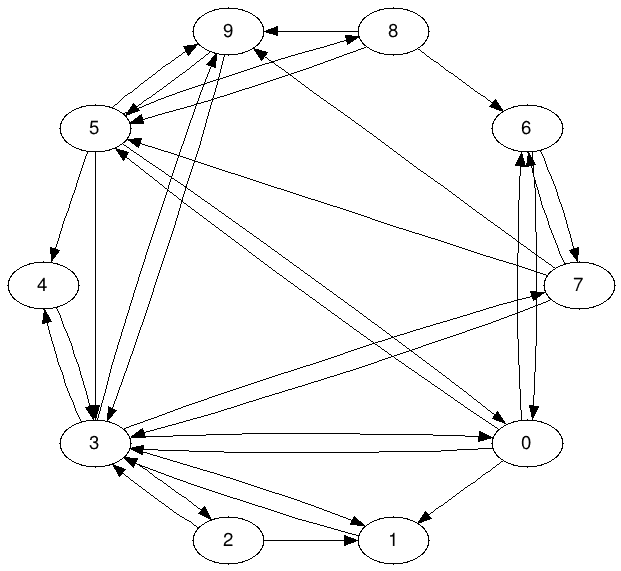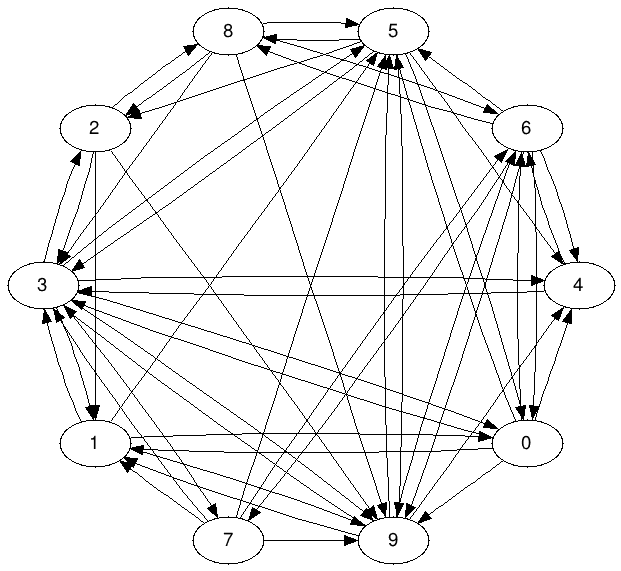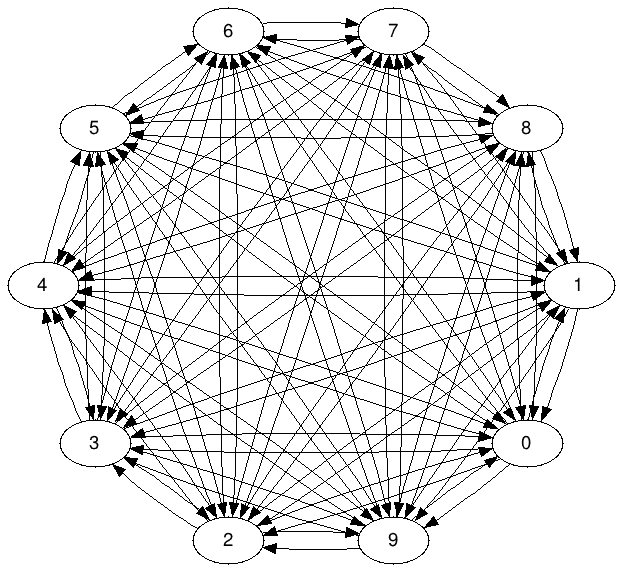n=10,p=0.1 n=10,p=0.3 n=10,p=0.5 n=10,p=0.9

The generation method has two phases:

• In the beginning, it is creating a random directed spanning tree to make sure that the graph is fully connected. (we can reach every node from other node)
• The second phase is the random arcs/edges addition phase, where random arcs/edges are added until the necessary connectivity rate has been reached

The framework implements measurement scenarios in the way that different algorithms can be compared to each other in the fairest way. We are only considering the calculation of the previous/distance vector, which is the core for the Dijkstra’s algorithm. For each experiment, the framework creates a random graph and runs 20 random vector generation from different origins. Only the runtime of the vector calculation is measured. This process is repeated 20 times and the 3 worst and best runtime has been dropped (to avoid outlier runtimes) and an average runtime is calculated from the remaining 14 runtimes.

### Results

The computer and runtime environment which was executing the tests has the following properties:
* Intel Core i7-4770K CPU
* 32 GB memory
* Java 1.7.0_51 JDK
* Windows 7 64bit SP1 OS

As described before, the framework has different implementations of the Dijkstra’s algorithm:

• Naive implementation
• Priority Queue with the built-in TreeSet binary heap
• Priority Queue with Neo4j’s Fibonacci heap
• Priority Queue with Nutch’s Fibonacci heap
• Priority Queue with Teneighty’s Fibonacci heap
• Priority Queue with Keithschwarz’s Fibonacci heap
• Unfortunately there were two implementations of the Fibonacci heap which produced unexpected exceptions during the tests
• Growing with the web’s Fibonacci heap
• Pengyifan’s Fibonacci heap

For the first run, I was curious about the implementations’ runtime on different graph sizes. The framework was executing the following file to find the right answer: GraphSizeAnalysisMain. It is measuring the runtime depends on different sizes (from n = 10 to n = 1000 in steps of 10) and connectivity (from p = 0.1 to p = 0.9 in steps of 0.2). The following figures contain the results: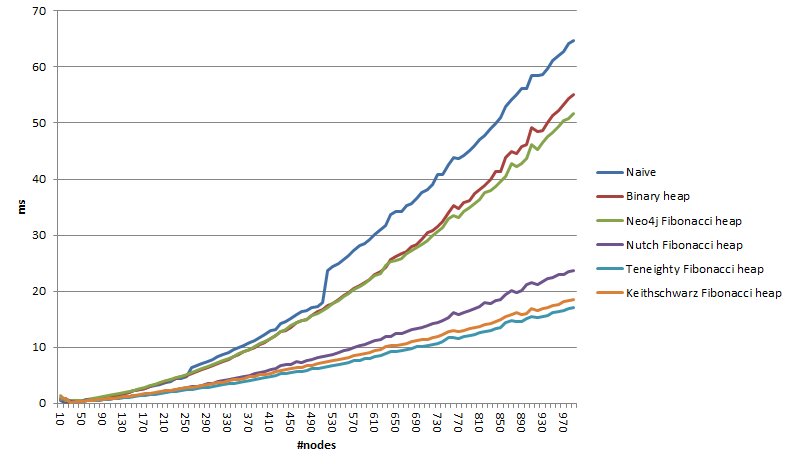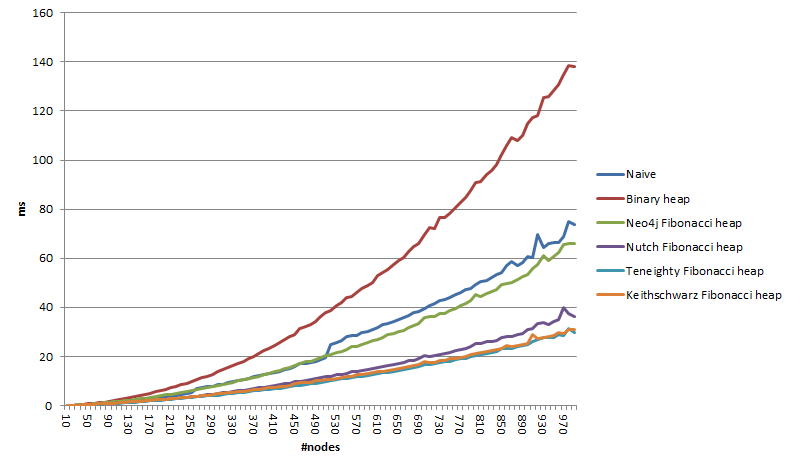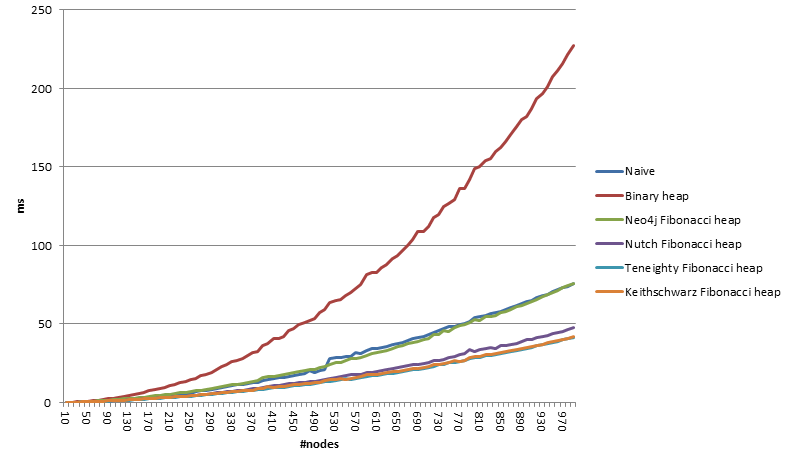Test case with p = 0.1 Test case with p = 0.3 Test case with p = 0.5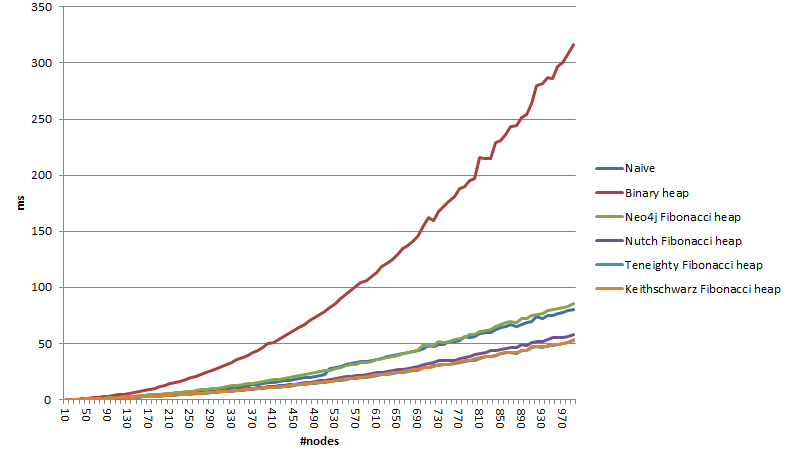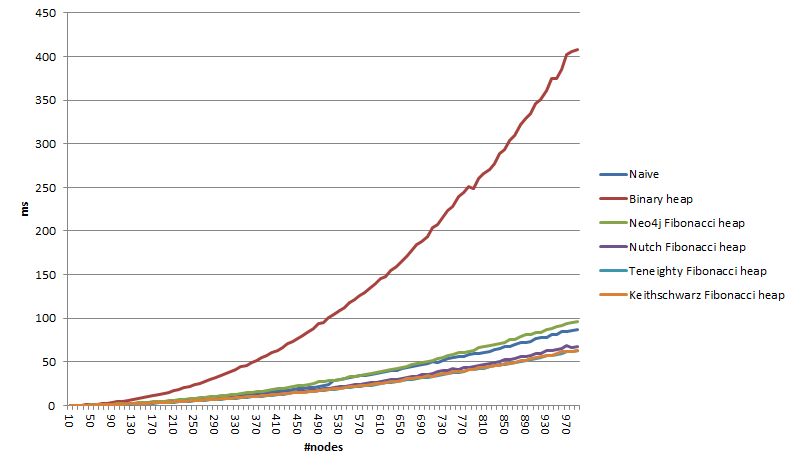Test case with p = 0.7 Test case with p = 0.9

The absolute winner was the Priority Queue version with Teneight’s Fibonacci heap and all Fibonacci heap Priority Queue implementation beats the other two. It is also interesting to see that in a spare graph, the naive implementation was overperformed by all the others implementation, but in a well connected graph, the naive implementation beats Priority Queue with binary heap implementation.

# Hello World

Hello World 🙂

This is going to be my first blog post ever 🙂 Hopefully I am going to have time for posting posts about interesting computer science and related topics. Currently I am pursuing my PhD at the University of York in the United Kingdom where I am participating on the Capacitie project where we (12 PhD students) are focusing in different aspects of pollution in the urban environment. If you are interested in my work, please visit the Projects or About pages where you can find out more information. Please feel free to comment my posts and feel free to contact me 🙂